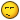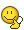BrainDen.com - Brain Teasers
• 0

# Matching values of Die throws pt. 3

## Recommended Posts

• 0

I'll be a smart a** and say it's seven minus the expected value of the lowest valued die.Seriously, I'll work on it.

##### Share on other sites

• 0

We seek the expected value of the highest individual score when n dice are thrown. We first find pn(k), the probability that the highest score is k. There are kn ways in which n dice can each show k or less.

##### Share on other sites

• 0

Suppose n fair 6-sided dice are rolled simultaneously. What is the expected value of the score on the highest valued die?

Let PN( i ) be the chance of having i as the highest score on simultaneous rolls of N dice. Obviously, PN( 0 ) = 0. The general expression for PN( i ) isNow that we have PN( i ), the expected value is simply

E = sum PN( i )* i for i from 1 to 6.

##### Share on other sites

• 0

7 - (1^n + 2^n + 3^n + 4^n + 5^n + 6^n)/6^n

##### Share on other sites

• 0

7 - (1^n + 2^n + 3^n + 4^n + 5^n + 6^n)/6^n

For the highest score to equal k, we must subtract those cases for which each die shows less than k; these number (k − 1)n.

So, k is the highest score in kn − (k − 1)n cases out of 6n.

In other words, pn(k), the probability that the highest individual score is k, is (kn − (k − 1)n)/6n.

The expected value, E(n), of the highest score is the sum, from k = 1 to 6, of k · pn(k).

##### Share on other sites

• 0

The table below shows E(n), correct to four decimal places, for various values of n, from 1 to 50. As expected intuitively, E(n) approaches a number, as n increases.

Expected values

n E(n)

1 3.5

2 4.4722

3 4.9583

4 5.2446

5 5.4309

6 5.5603

7 5.6541

8 5.7244

9 5.7782

10 5.8202

20 5.9736

30 5.9958

40 5.9993

50 5.9999

##### Share on other sites

• 0

7 - (1^n + 2^n + 3^n + 4^n + 5^n + 6^n)/6^n

For the highest score to equal k, we must subtract those cases for which each die shows less than k; these number (k − 1)n.

So, k is the highest score in kn − (k − 1)n cases out of 6n.

In other words, pn(k), the probability that the highest individual score is k, is (kn − (k − 1)n)/6n.

The expected value, E(n), of the highest score is the sum, from k = 1 to 6, of k · pn(k).

That's the obvious and natural approach. And that's exactly how I got the formula.

When you say "close but", you mean that the formula is wrong?

##### Share on other sites

• 0

it wouldn't be the first time I made a mistake on one of my own problems, but yes, i derived a slightly different answer.

##### Share on other sites

• 0

it wouldn't be the first time I made a mistake on one of my own problems, but yes, i derived a slightly different answer.

I applied witzar's formula to various different N, and I reproduced the same exact table that you gave in here

The table below shows E(n), correct to four decimal places, for various values of n, from 1 to 50. As expected intuitively, E(n) approaches a number, as n increases.

Expected values

n E(n)

1 3.5

2 4.4722

3 4.9583

4 5.2446

5 5.4309

6 5.5603

7 5.6541

8 5.7244

9 5.7782

10 5.8202

20 5.9736

30 5.9958

40 5.9993

50 5.9999

##### Share on other sites

• 0

There are kn ways in which n dice can each show k or less.
For the highest score to equal k, we must subtract those cases for which each die shows less than k; these number (k − 1)n.
So, k is the highest score in kn − (k − 1)n cases out of 6n.
In other words, pn(k), the probability that the highest individual score is k, is (kn − (k − 1)n)/6n.

The expected value, E(n), of the highest score is the sum, from k = 1 to 6, of k · pn(k).

Hence E(n) = [6(6n−5n) + 5(5n−4n) + 4(4n−3n) + 3(3n−2n) + 2(2n−1n) + 1(1n−0n)]/6n. = 6 − (1n + 2n + 3n + 4n + 5n)/6n.

##### Share on other sites

• 0

There are kn ways in which n dice can each show k or less.

For the highest score to equal k, we must subtract those cases for which each die shows less than k; these number (k − 1)n.

So, k is the highest score in kn − (k − 1)n cases out of 6n.

In other words, pn(k), the probability that the highest individual score is k, is (kn − (k − 1)n)/6n.

The expected value, E(n), of the highest score is the sum, from k = 1 to 6, of k · pn(k).

Hence E(n) = [6(6n−5n) + 5(5n−4n) + 4(4n−3n) + 3(3n−2n) + 2(2n−1n) + 1(1n−0n)]/6n. = 6 − (1n + 2n + 3n + 4n + 5n)/6n.

Nevermind, they are equivalent. See witzar's

##### Share on other sites

• 0I concede to Witzar.

There are kn ways in which n dice can each show k or less.
For the highest score to equal k, we must subtract those cases for which each die shows less than k; these number (k − 1)n.
So, k is the highest score in kn − (k − 1)n cases out of 6n.
In other words, pn(k), the probability that the highest individual score is k, is (kn − (k − 1)n)/6n.

The expected value, E(n), of the highest score is the sum, from k = 1 to 6, of k · pn(k).

Hence E(n) = [6(6n−5n) + 5(5n−4n) + 4(4n−3n) + 3(3n−2n) + 2(2n−1n) + 1(1n−0n)]/6n. = 6 − (1n + 2n + 3n + 4n + 5n)/6n.

Let's take a look at the highlighted equation above

Let's play with only 1 die so n = 1. So the expected value of the highest number is simply (1 + 2 + 3 + 4 + 5 + 6)/6 = 3.5

But if you plug it in the above formula, you'll get a different value for the expected face number

6 - (1 + 2 + 3 + 4 + 5 + 6)/6 = 6 - 3.5 = 2.5

##### Share on other sites

• 0

There are kn ways in which n dice can each show k or less.

For the highest score to equal k, we must subtract those cases for which each die shows less than k; these number (k − 1)n.

So, k is the highest score in kn − (k − 1)n cases out of 6n.

In other words, pn(k), the probability that the highest individual score is k, is (kn − (k − 1)n)/6n.

The expected value, E(n), of the highest score is the sum, from k = 1 to 6, of k · pn(k).

Hence E(n) = [6(6n−5n) + 5(5n−4n) + 4(4n−3n) + 3(3n−2n) + 2(2n−1n) + 1(1n−0n)]/6n. = 6 − (1n + 2n + 3n + 4n + 5n)/6n.

7 - (1^n + 2^n + 3^n + 4^n + 5^n + 6^n)/6^n =

= 6 + 6^n/6^n - (1^n + 2^n + 3^n + 4^n + 5^n + 6^n)/6^n =

= 6 - (1^n + 2^n + 3^n + 4^n + 5^n)/6^n

##### Share on other sites

• 0

There are kn ways in which n dice can each show k or less.

For the highest score to equal k, we must subtract those cases for which each die shows less than k; these number (k − 1)n.

So, k is the highest score in kn − (k − 1)n cases out of 6n.

In other words, pn(k), the probability that the highest individual score is k, is (kn − (k − 1)n)/6n.

The expected value, E(n), of the highest score is the sum, from k = 1 to 6, of k · pn(k).

Hence E(n) = [6(6n−5n) + 5(5n−4n) + 4(4n−3n) + 3(3n−2n) + 2(2n−1n) + 1(1n−0n)]/6n. = 6 − (1n + 2n + 3n + 4n + 5n)/6n.

7 - (1^n + 2^n + 3^n + 4^n + 5^n + 6^n)/6^n =

= 6 + 6^n/6^n - (1^n + 2^n + 3^n + 4^n + 5^n + 6^n)/6^n =

= 6 - (1^n + 2^n + 3^n + 4^n + 5^n)/6^n

if they are equivalent then why don't they produce the same expected values as Bushindo pointed out.

##### Share on other sites

• 0

There are kn ways in which n dice can each show k or less.

For the highest score to equal k, we must subtract those cases for which each die shows less than k; these number (k − 1)n.

So, k is the highest score in kn − (k − 1)n cases out of 6n.

In other words, pn(k), the probability that the highest individual score is k, is (kn − (k − 1)n)/6n.

The expected value, E(n), of the highest score is the sum, from k = 1 to 6, of k · pn(k).

Hence E(n) = [6(6n−5n) + 5(5n−4n) + 4(4n−3n) + 3(3n−2n) + 2(2n−1n) + 1(1n−0n)]/6n. = 6 − (1n + 2n + 3n + 4n + 5n)/6n.

7 - (1^n + 2^n + 3^n + 4^n + 5^n + 6^n)/6^n =

= 6 + 6^n/6^n - (1^n + 2^n + 3^n + 4^n + 5^n + 6^n)/6^n =

= 6 - (1^n + 2^n + 3^n + 4^n + 5^n)/6^n

if they are equivalent then why don't they produce the same expected values as Bushindo pointed out.

I misread the equation. Yours was

6 − (1n + 2n + 3n + 4n + 5n)/6n.

6 − (1n + 2n + 3n + 4n + 5n + 6n) /6n.

Mea culpa =)

##### Share on other sites

• 0

if they are equivalent then why don't they produce the same expected values as Bushindo pointed out.

They do of course. Bushindo made a silly error evaluating your formula.
##### Share on other sites

• 0

I have a question. What will be the expected value if only 1 dice is rolled?

##### Share on other sites

• 0

Ok thanks. I didnt realise expected value may be a fraction.

## Join the conversation

You can post now and register later. If you have an account, sign in now to post with your account.×   Pasted as rich text.   Paste as plain text instead

Only 75 emoji are allowed.

×   Your previous content has been restored.   Clear editor

×   You cannot paste images directly. Upload or insert images from URL.